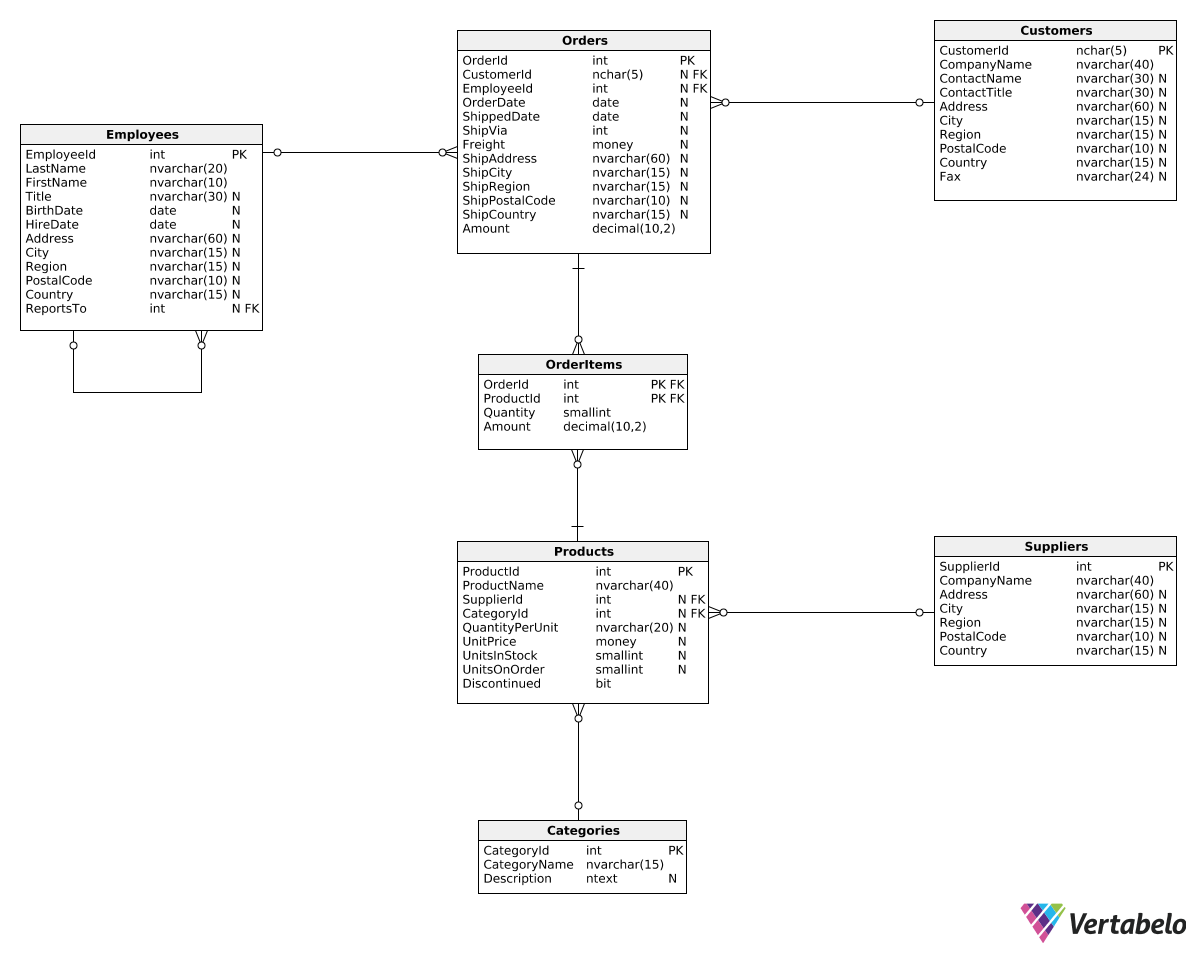Deals Of The Week - hours only!Up to 80% off on all courses and bundles.-Close
Introduction
Total revenue for multiple periods
Calculating deltas
Creating "revenue in quarters" reports
Summary

## Instruction

Awesome! We can also express the delta between two periods as a percentage. Look at the following query:

SELECT
DATEPART(Year, OrderDate) AS RevenueYear,
SUM(Amount) AS TotalRevenue,
ROUND(
100 *
(SUM(Amount) - LAG(SUM(Amount), 1)
OVER (ORDER BY DATEPART(Year, OrderDate)))
/ CAST(LAG(SUM(Amount), 1)
OVER (ORDER BY DATEPART(Year, OrderDate)) AS decimal(10,2)),
2) AS DeltaPercentage
FROM Orders
GROUP BY DATEPART(Year, OrderDate)
ORDER BY DATEPART(Year, OrderDate);


Here is the result:

RevenueYear TotalRevenue DeltaPercentage
2016 218600.23 NULL
2017 655506.15 199.87
2018 273379.76 -58.29

This time, the calculation in the last column is a bit more complicated:

1. We divide the change between the current and previous period by the revenue value from the previous period.
2. To avoid integer division, we cast the denominator to a decimal type with CAST(SUM(Amount) AS decimal(10,2))). For larger numbers, you can of course increase the first argument of decimal(10,2).
3. We multiply the division result by 100 to get the percentage value.
4. We round the percentage to two decimal places using the ROUND(value, 2) function.

## Exercise

The template query contains the code from the previous example. Modify it to show the revenue change as a percentage that's rounded to three decimal places.

Here's how you can count the revenue change as a percentage:

\frac{\textrm{total amount} - \textrm{total amount from preceding quarters}}{\textrm{total amount from preceding quarters}} \cdot 100

Remember to round the result to three decimal places.

### Stuck? Here's a hint!

Use the following expression to calculate the last column:

ROUND(
100 *
(SUM(Amount) - LAG(SUM(Amount), 1)
OVER (ORDER BY DATEPART(Year, OrderDate),
DATEPART(Quarter, OrderDate)))
/ CAST(LAG(SUM(Amount), 1)
OVER (ORDER BY DATEPART(Year, OrderDate),
DATEPART(Quarter, OrderDate)) AS decimal(10,2)),
3) AS Delta